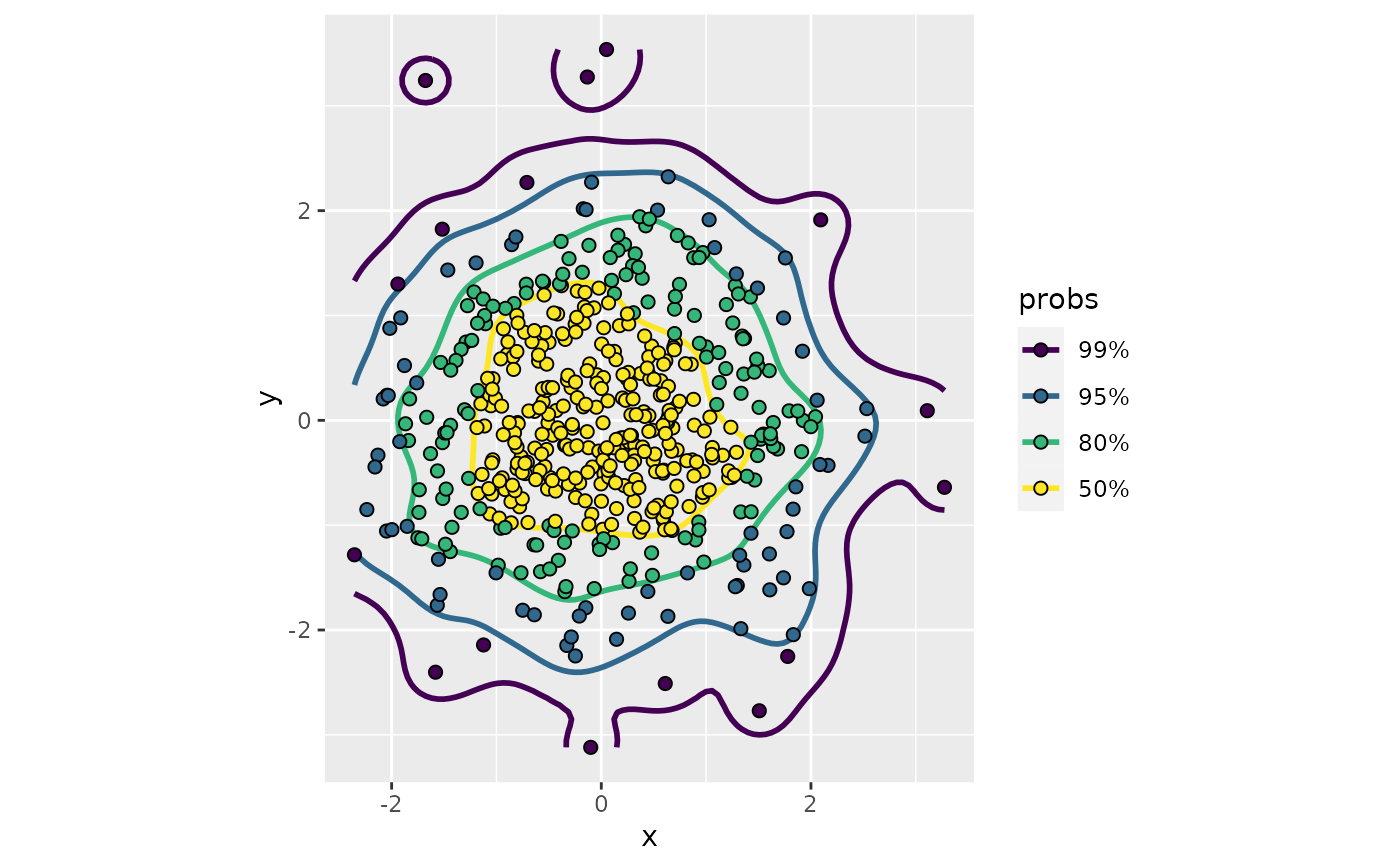Perform 2D density estimation, compute the resulting highest density regions (HDRs), and plot the provided data as a scatterplot with points colored according to their corresponding HDR

## Usage

stat_hdr_points(
mapping = NULL,
data = NULL,
geom = "point",
position = "identity",
...,
method = "kde",
probs = c(0.99, 0.95, 0.8, 0.5),
bins = NULL,
n = 100,
xlim = NULL,
ylim = NULL,
nudgex = "none",
nudgey = "none",
smooth = FALSE,
h = NULL,
na.rm = FALSE,
show.legend = NA,
inherit.aes = TRUE
)

geom_hdr_points(
mapping = NULL,
data = NULL,
stat = "hdr_points",
position = "identity",
...,
method = "kde",
probs = c(0.99, 0.95, 0.8, 0.5),
bins = NULL,
n = 100,
xlim = NULL,
ylim = NULL,
nudgex = "none",
nudgey = "none",
smooth = FALSE,
h = NULL,
na.rm = FALSE,
show.legend = NA,
inherit.aes = TRUE
)

## Arguments

mapping

Set of aesthetic mappings created by aes() or aes_(). If specified and inherit.aes = TRUE (the default), it is combined with the default mapping at the top level of the plot. You must supply mapping if there is no plot mapping.

data

The data to be displayed in this layer. There are three options:

If NULL, the default, the data is inherited from the plot data as specified in the call to ggplot().

A data.frame, or other object, will override the plot data. All objects will be fortified to produce a data frame. See fortify() for which variables will be created.

A function will be called with a single argument, the plot data. The return value must be a data.frame, and will be used as the layer data. A function can be created from a formula (e.g. ~ head(.x, 10)).

geom

The geometric object to use display the data

position

Position adjustment, either as a string, or the result of a call to a position adjustment function.

...

Other arguments passed on to layer(). These are often aesthetics, used to set an aesthetic to a fixed value, like colour = "red" or size = 3. They may also be parameters to the paired geom/stat.

method

Density estimator to use, accepts character vector: "kde", "histogram", "freqpoly", or "mvnorm".

probs

Probabilities to compute highest density regions for.

bins

Number of bins along each axis for histogram and frequency polygon estimators. Either a vector of length 2 or a scalar value which is recycled for both dimensions. Defaults to normal reference rule (Scott, pg 87).

n

Number of grid points in each direction.

xlim, ylim

Range to compute and draw regions. If NULL, defaults to range of data.

nudgex

Horizontal rule for choosing witness points for smoothed histogram method, accepts character vector: "left", "none", "right".

nudgey

Vertical rule for choosing witness points for smoothed histogram method, accepts character vector: "down", "none", "up".

smooth

If TRUE, HDRs computed by the "histogram" method are smoothed.

A multiplicative bandwidth adjustment to be used if 'h' is 'NULL'. This makes it possible to adjust the bandwidth while still using the a bandwidth estimator. For example, adjust = 1/2 means use half of the default bandwidth.

h

Bandwidth (vector of length two). If NULL, estimated using MASS::bandwidth.nrd().

na.rm

If FALSE, the default, missing values are removed with a warning. If TRUE, missing values are silently removed.

show.legend

logical. Should this layer be included in the legends? NA, the default, includes if any aesthetics are mapped. FALSE never includes, and TRUE always includes. It can also be a named logical vector to finely select the aesthetics to display.

inherit.aes

If FALSE, overrides the default aesthetics, rather than combining with them. This is most useful for helper functions that define both data and aesthetics and shouldn't inherit behaviour from the default plot specification, e.g. borders().

stat

The statistical transformation to use on the data for this layer, as a string.

## Aesthetics

geom_hdr_points understands the following aesthetics (required aesthetics are in bold):

• x

• y

• alpha

• color

• fill

• group

• linetype

• size

• subgroup

## Computed variables

probs

The probability associated with the highest density region, specified by probs.

## Examples


# basic simulated data with bivariate normal data and various methods
# (note: code is commented out in this file to save cran check time)
df <- data.frame(x = rnorm(500), y = rnorm(500))
p <- ggplot(df, aes(x, y)) + coord_equal()
p + geom_hdr_points()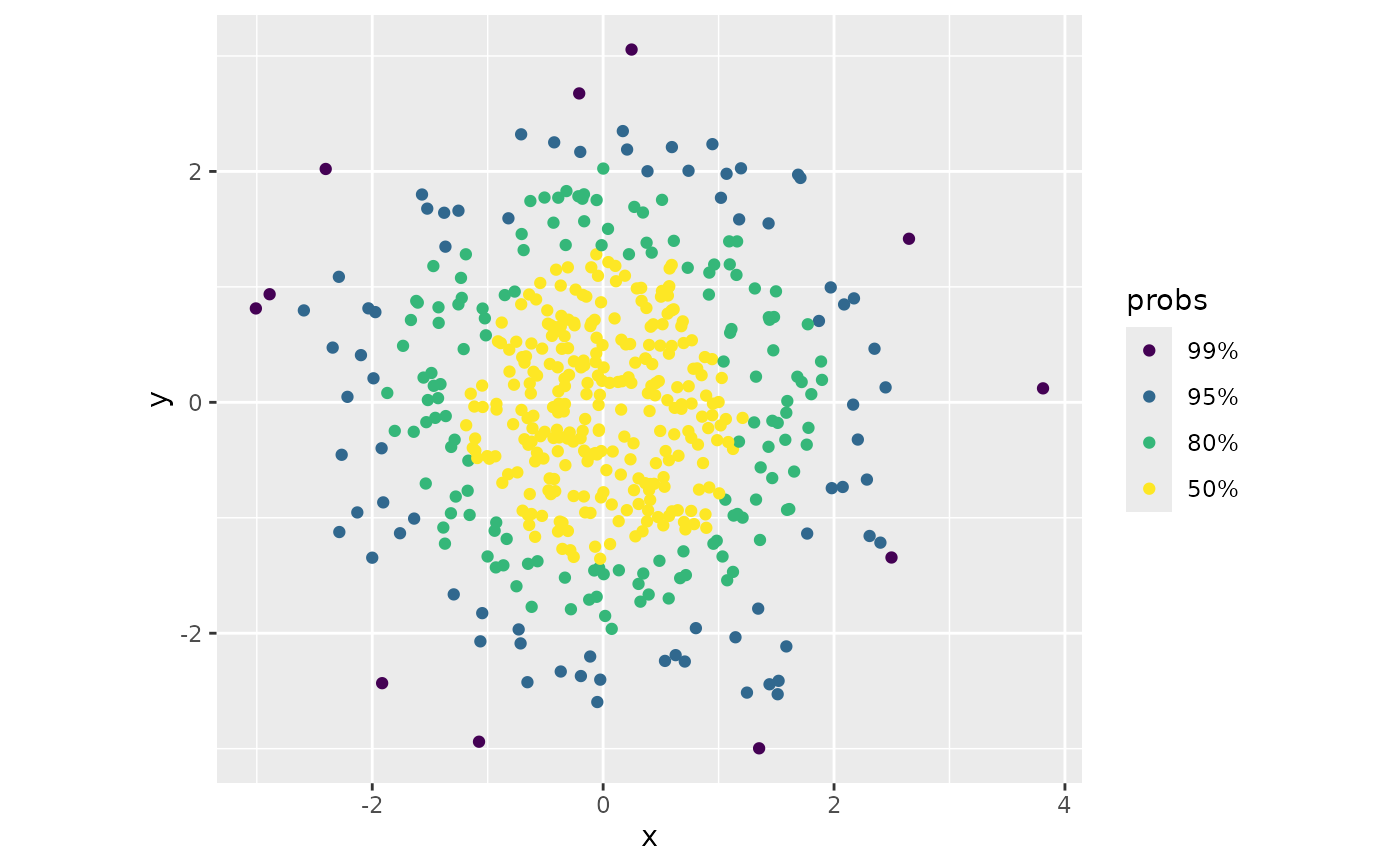p + geom_hdr_points(method = "mvnorm")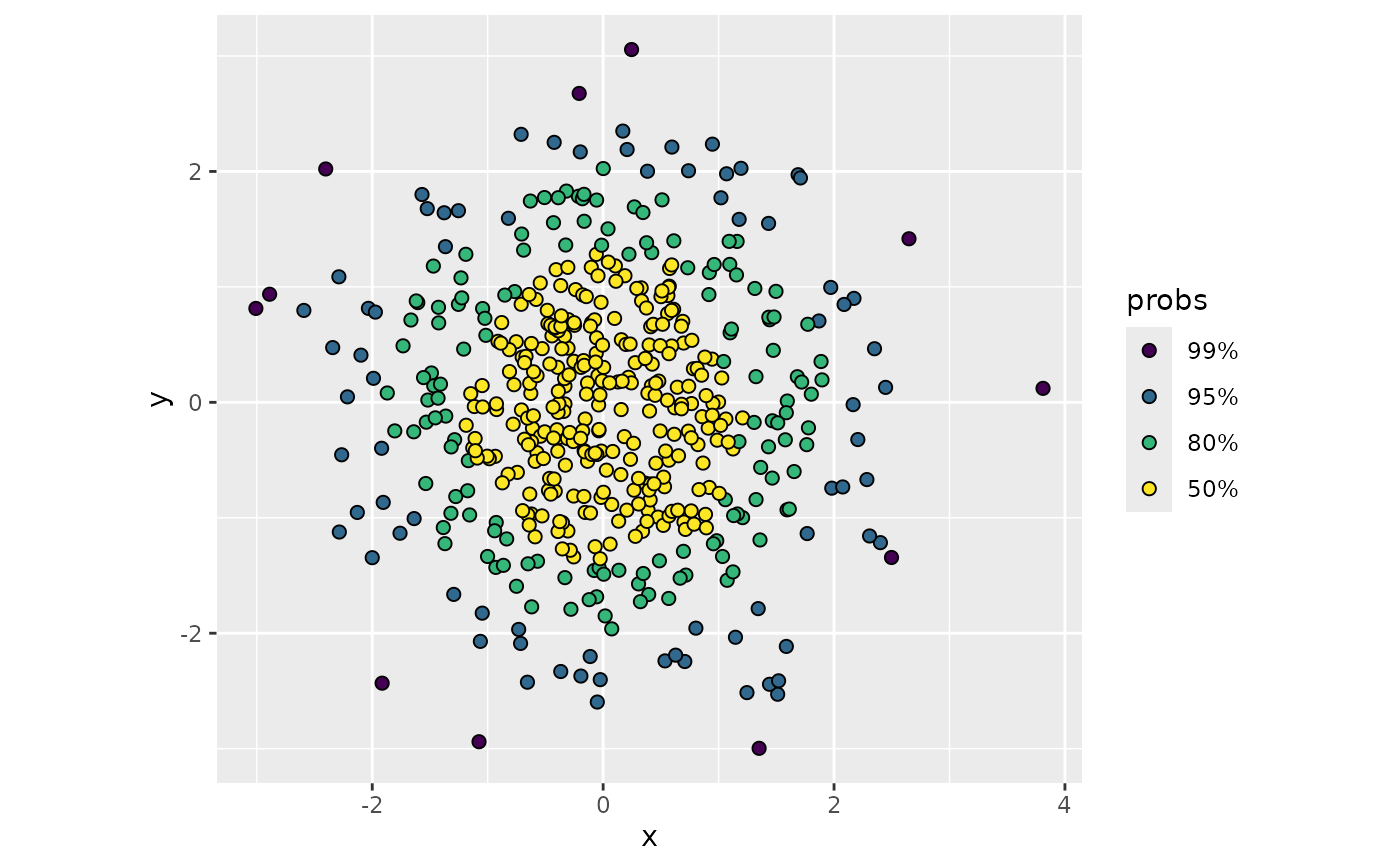p + geom_hdr_points(method = "freqpoly")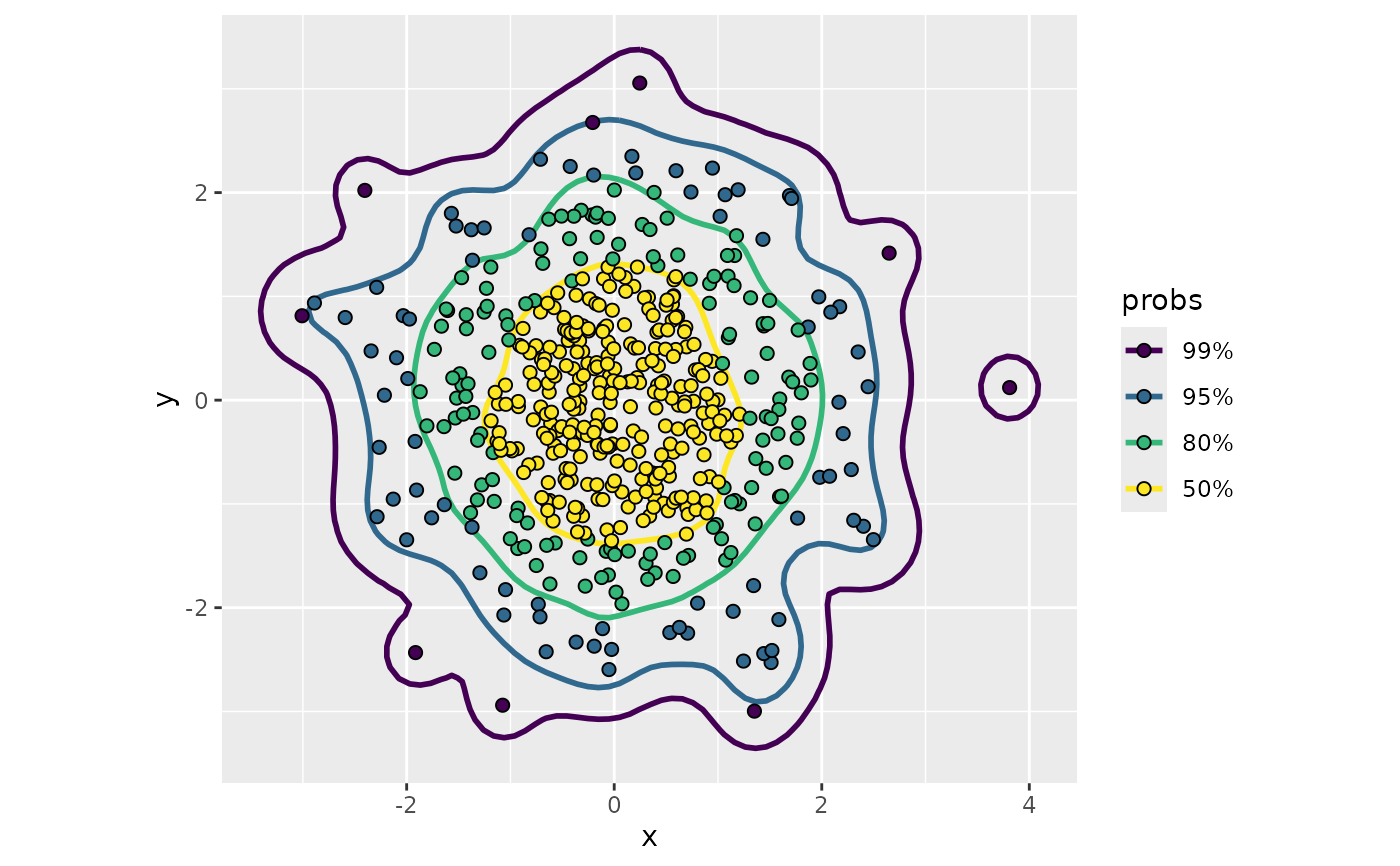# p + geom_hdr_points(method = "histogram")

# setting aes(fill = after_stat(probs)), color = "black", and
# shape = 21 helps alleviate overplotting:
p + geom_hdr_points(aes(fill = after_stat(probs)), color = "black", shape = 21, size = 2)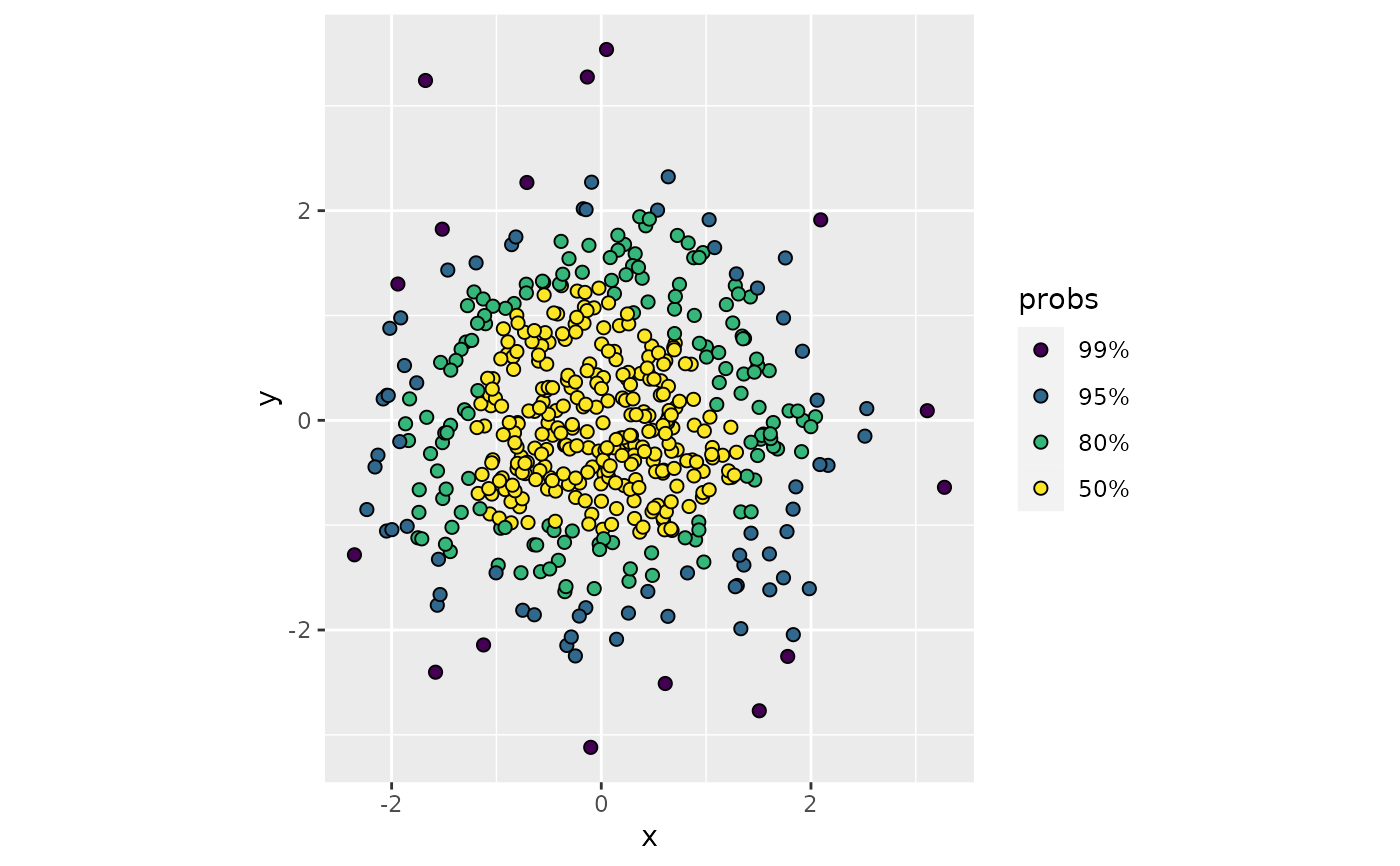# also works well with geom_hdr_lines():
p + geom_hdr_lines(aes(color = after_stat(probs)), alpha = 1) +
geom_hdr_points(aes(fill = after_stat(probs)), color = "black", shape = 21, size = 2)